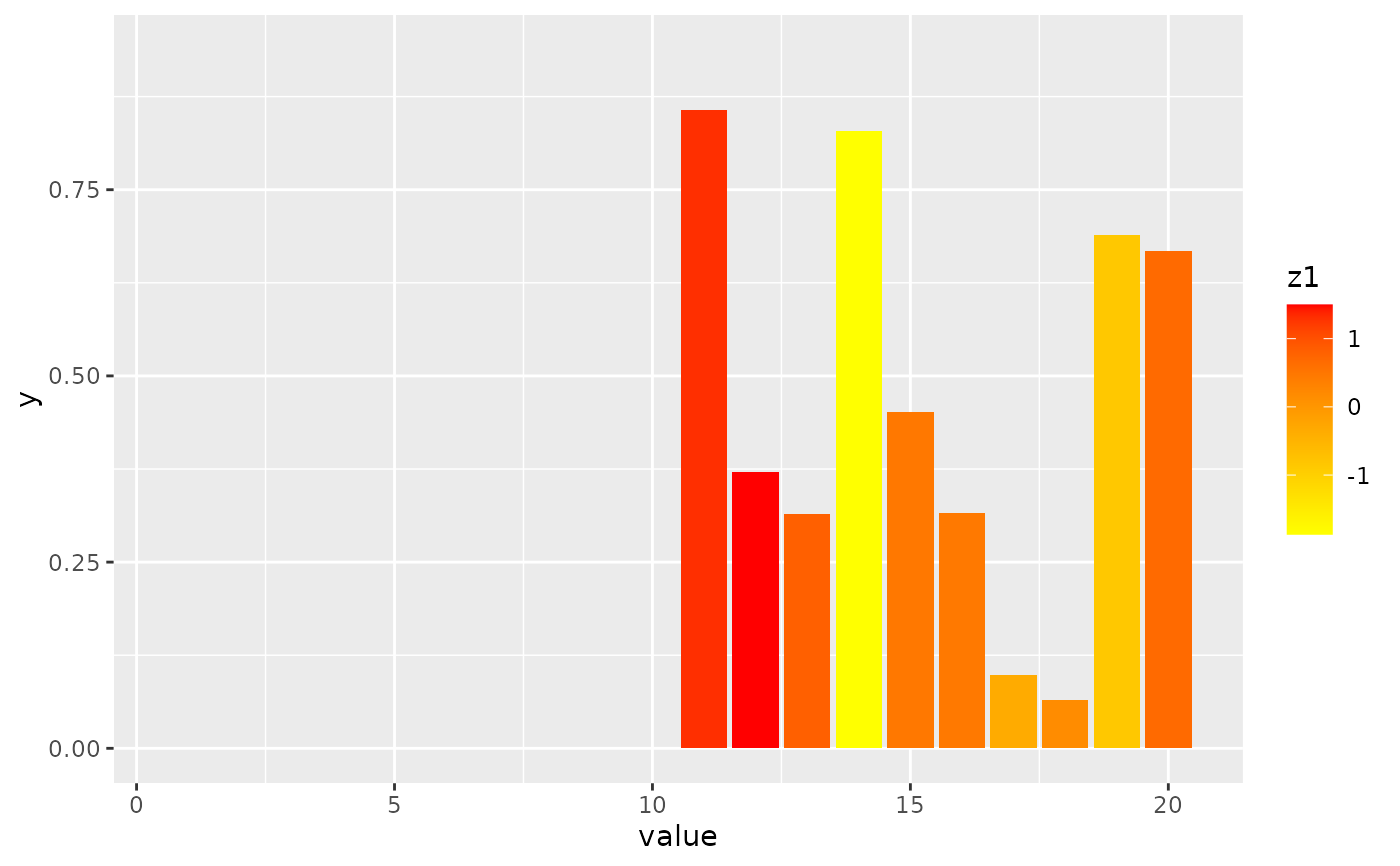scale_*_gradient creates a two colour gradient (low-high), scale_*_gradient2 creates a diverging colour gradient (low-mid-high), scale_*_gradientn creates a n-colour gradient. For binned variants of these scales, see the color steps scales.

## Usage

scale_colour_gradient(
...,
low = "#132B43",
high = "#56B1F7",
space = "Lab",
na.value = "grey50",
guide = "colourbar",
aesthetics = "colour"
)

...,
low = "#132B43",
high = "#56B1F7",
space = "Lab",
na.value = "grey50",
guide = "colourbar",
aesthetics = "fill"
)

...,
low = muted("red"),
mid = "white",
high = muted("blue"),
midpoint = 0,
space = "Lab",
na.value = "grey50",
guide = "colourbar",
aesthetics = "colour"
)

...,
low = muted("red"),
mid = "white",
high = muted("blue"),
midpoint = 0,
space = "Lab",
na.value = "grey50",
guide = "colourbar",
aesthetics = "fill"
)

...,
colours,
values = NULL,
space = "Lab",
na.value = "grey50",
guide = "colourbar",
aesthetics = "colour",
colors
)

...,
colours,
values = NULL,
space = "Lab",
na.value = "grey50",
guide = "colourbar",
aesthetics = "fill",
colors
)

## Arguments

...

Arguments passed on to continuous_scale

scale_name

The name of the scale that should be used for error messages associated with this scale.

palette

A palette function that when called with a numeric vector with values between 0 and 1 returns the corresponding output values (e.g., scales::area_pal()).

name

The name of the scale. Used as the axis or legend title. If waiver(), the default, the name of the scale is taken from the first mapping used for that aesthetic. If NULL, the legend title will be omitted.

breaks

One of:

• NULL for no breaks

• waiver() for the default breaks computed by the transformation object

• A numeric vector of positions

• A function that takes the limits as input and returns breaks as output (e.g., a function returned by scales::extended_breaks()). Also accepts rlang lambda function notation.

minor_breaks

One of:

• NULL for no minor breaks

• waiver() for the default breaks (one minor break between each major break)

• A numeric vector of positions

• A function that given the limits returns a vector of minor breaks. Also accepts rlang lambda function notation.

n.breaks

An integer guiding the number of major breaks. The algorithm may choose a slightly different number to ensure nice break labels. Will only have an effect if breaks = waiver(). Use NULL to use the default number of breaks given by the transformation.

labels

One of:

• NULL for no labels

• waiver() for the default labels computed by the transformation object

• A character vector giving labels (must be same length as breaks)

• A function that takes the breaks as input and returns labels as output. Also accepts rlang lambda function notation.

limits

One of:

• NULL to use the default scale range

• A numeric vector of length two providing limits of the scale. Use NA to refer to the existing minimum or maximum

• A function that accepts the existing (automatic) limits and returns new limits. Also accepts rlang lambda function notation. Note that setting limits on positional scales will remove data outside of the limits. If the purpose is to zoom, use the limit argument in the coordinate system (see coord_cartesian()).

rescaler

A function used to scale the input values to the range [0, 1]. This is always scales::rescale(), except for diverging and n colour gradients (i.e., scale_colour_gradient2(), scale_colour_gradientn()). The rescaler is ignored by position scales, which always use scales::rescale(). Also accepts rlang lambda function notation.

oob

One of:

• Function that handles limits outside of the scale limits (out of bounds). Also accepts rlang lambda function notation.

• The default (scales::censor()) replaces out of bounds values with NA.

• scales::squish() for squishing out of bounds values into range.

• scales::squish_infinite() for squishing infinite values into range.

trans

For continuous scales, the name of a transformation object or the object itself. Built-in transformations include "asn", "atanh", "boxcox", "date", "exp", "hms", "identity", "log", "log10", "log1p", "log2", "logit", "modulus", "probability", "probit", "pseudo_log", "reciprocal", "reverse", "sqrt" and "time".

A transformation object bundles together a transform, its inverse, and methods for generating breaks and labels. Transformation objects are defined in the scales package, and are called <name>_trans (e.g., scales::boxcox_trans()). You can create your own transformation with scales::trans_new().

expand

For position scales, a vector of range expansion constants used to add some padding around the data to ensure that they are placed some distance away from the axes. Use the convenience function expansion() to generate the values for the expand argument. The defaults are to expand the scale by 5% on each side for continuous variables, and by 0.6 units on each side for discrete variables.

position

For position scales, The position of the axis. left or right for y axes, top or bottom for x axes.

super

The super class to use for the constructed scale

low, high

Colours for low and high ends of the gradient.

space

colour space in which to calculate gradient. Must be "Lab" - other values are deprecated.

na.value

Colour to use for missing values

guide

Type of legend. Use "colourbar" for continuous colour bar, or "legend" for discrete colour legend.

aesthetics

Character string or vector of character strings listing the name(s) of the aesthetic(s) that this scale works with. This can be useful, for example, to apply colour settings to the colour and fill aesthetics at the same time, via aesthetics = c("colour", "fill").

mid

colour for mid point

midpoint

The midpoint (in data value) of the diverging scale. Defaults to 0.

colours, colors

Vector of colours to use for n-colour gradient.

values

if colours should not be evenly positioned along the gradient this vector gives the position (between 0 and 1) for each colour in the colours vector. See rescale() for a convenience function to map an arbitrary range to between 0 and 1.

## Details

Default colours are generated with munsell and mnsl(c("2.5PB 2/4", "2.5PB 7/10")). Generally, for continuous colour scales you want to keep hue constant, but vary chroma and luminance. The munsell package makes this easy to do using the Munsell colour system.

scales::seq_gradient_pal() for details on underlying palette, scale_colour_steps() for binned variants of these scales.

Other colour scales: scale_alpha(), scale_colour_brewer(), scale_colour_continuous(), scale_colour_grey(), scale_colour_hue(), scale_colour_steps(), scale_colour_viridis_d()

## Examples

df <- data.frame(
x = runif(100),
y = runif(100),
z1 = rnorm(100),
z2 = abs(rnorm(100))
)

df_na <- data.frame(
value = seq(1, 20),
x = runif(20),
y = runif(20),
z1 = c(rep(NA, 10), rnorm(10))
)

# Default colour scale colours from light blue to dark blue
ggplot(df, aes(x, y)) +
geom_point(aes(colour = z2))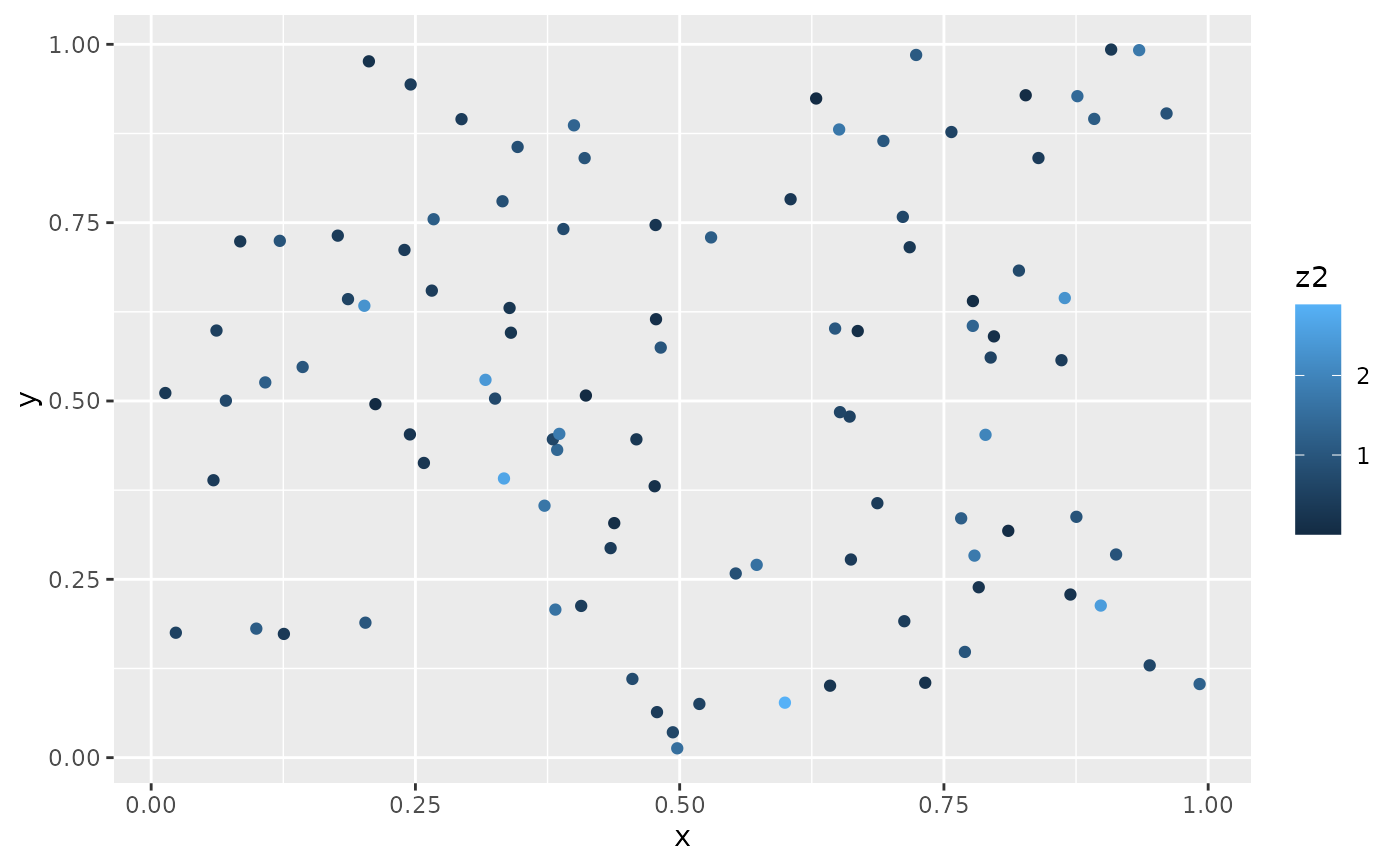# For diverging colour scales use gradient2
ggplot(df, aes(x, y)) +
geom_point(aes(colour = z1)) +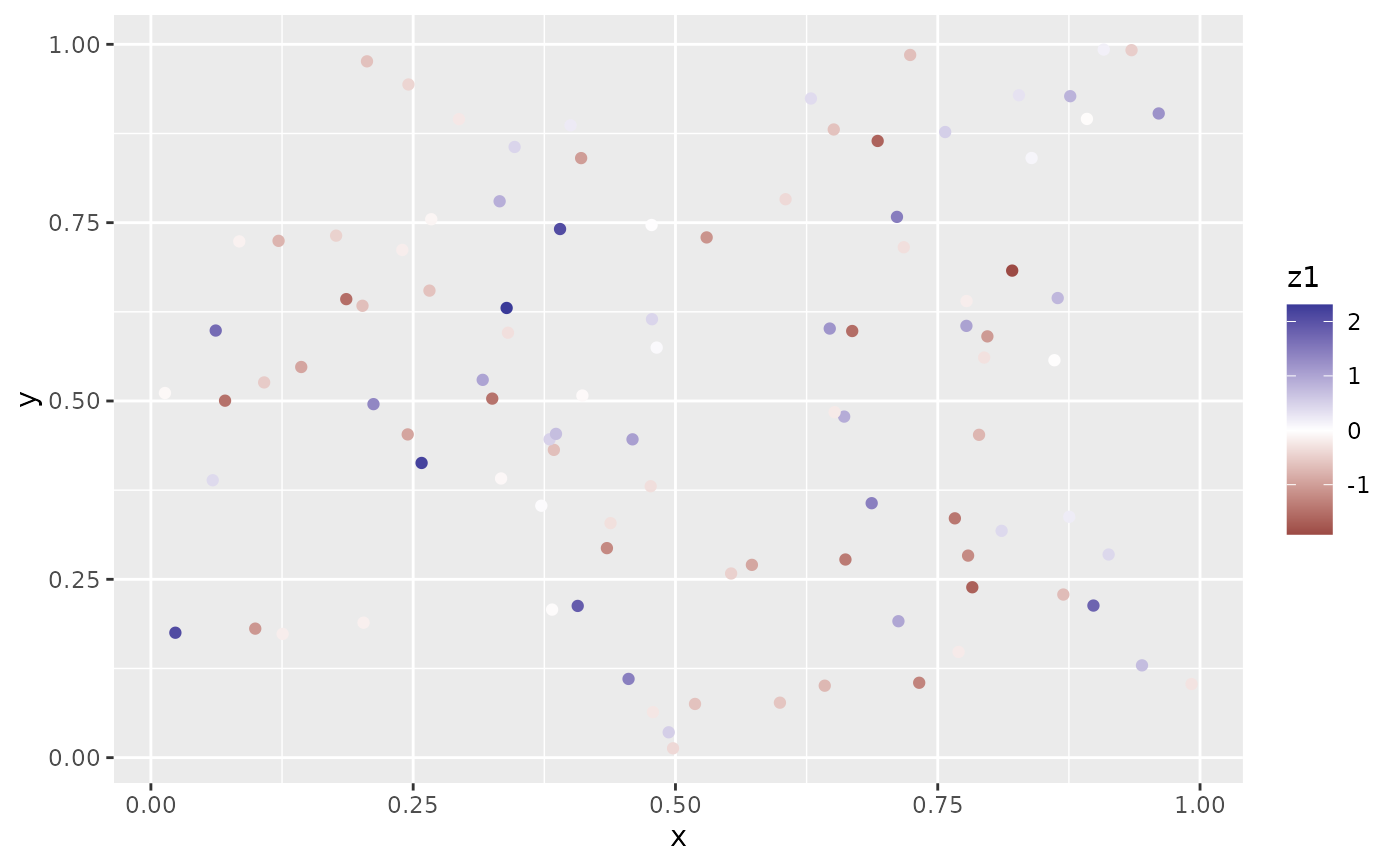# Use your own colour scale with gradientn
ggplot(df, aes(x, y)) +
geom_point(aes(colour = z1)) +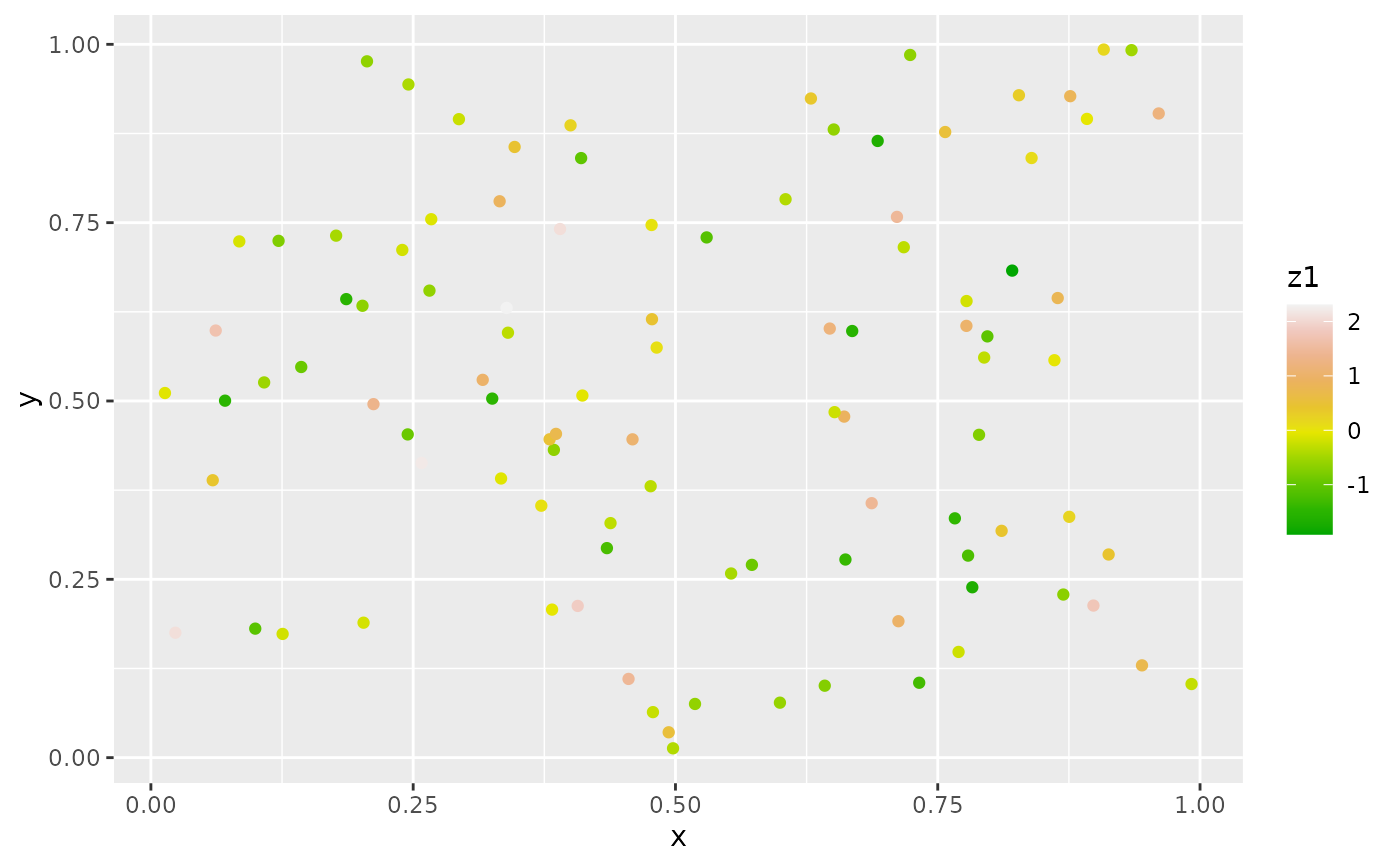# Equivalent fill scales do the same job for the fill aesthetic
ggplot(faithfuld, aes(waiting, eruptions)) +
geom_raster(aes(fill = density)) +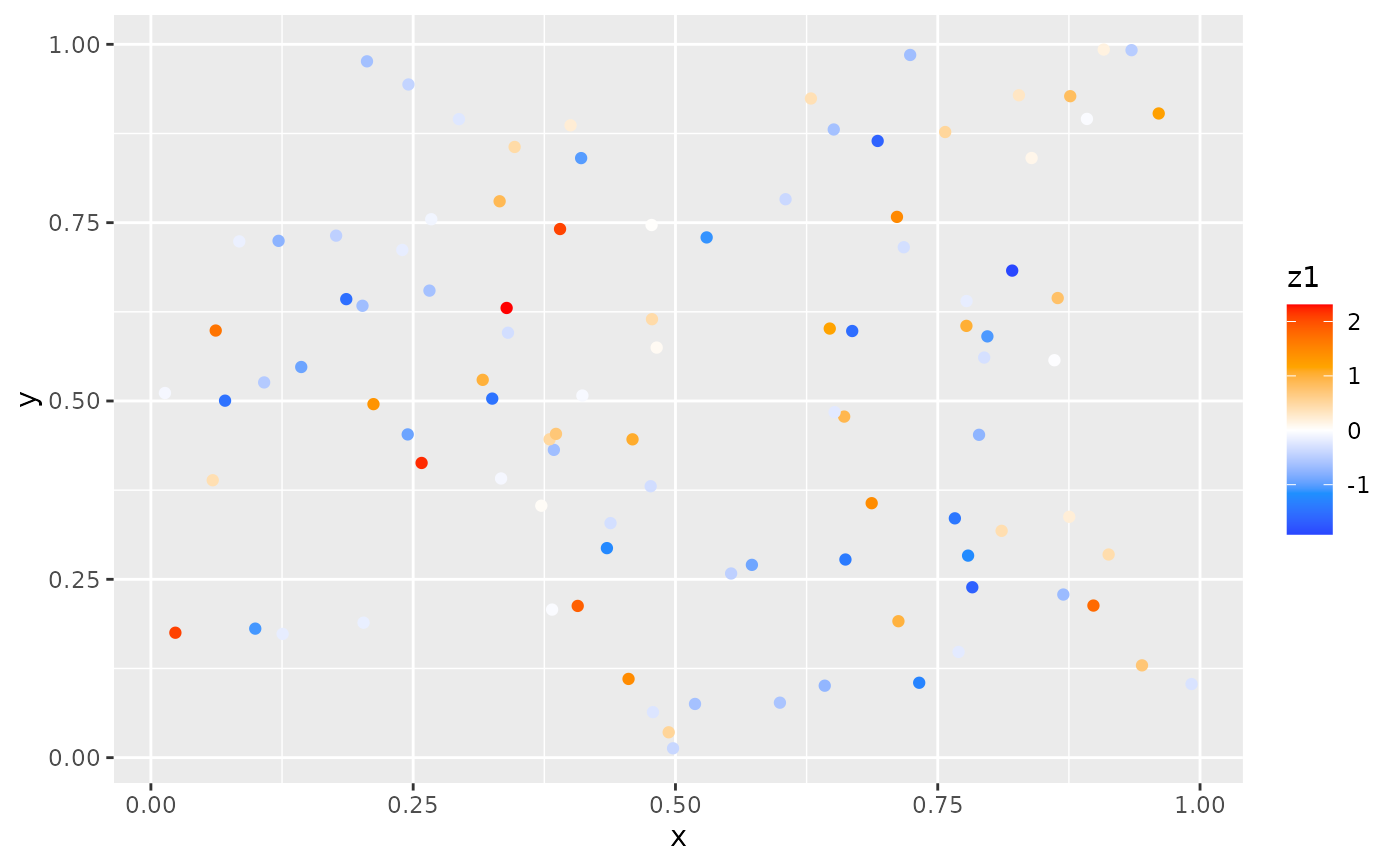# Adjust colour choices with low and high
ggplot(df, aes(x, y)) +
geom_point(aes(colour = z2)) +
scale_colour_gradient(low = "white", high = "black")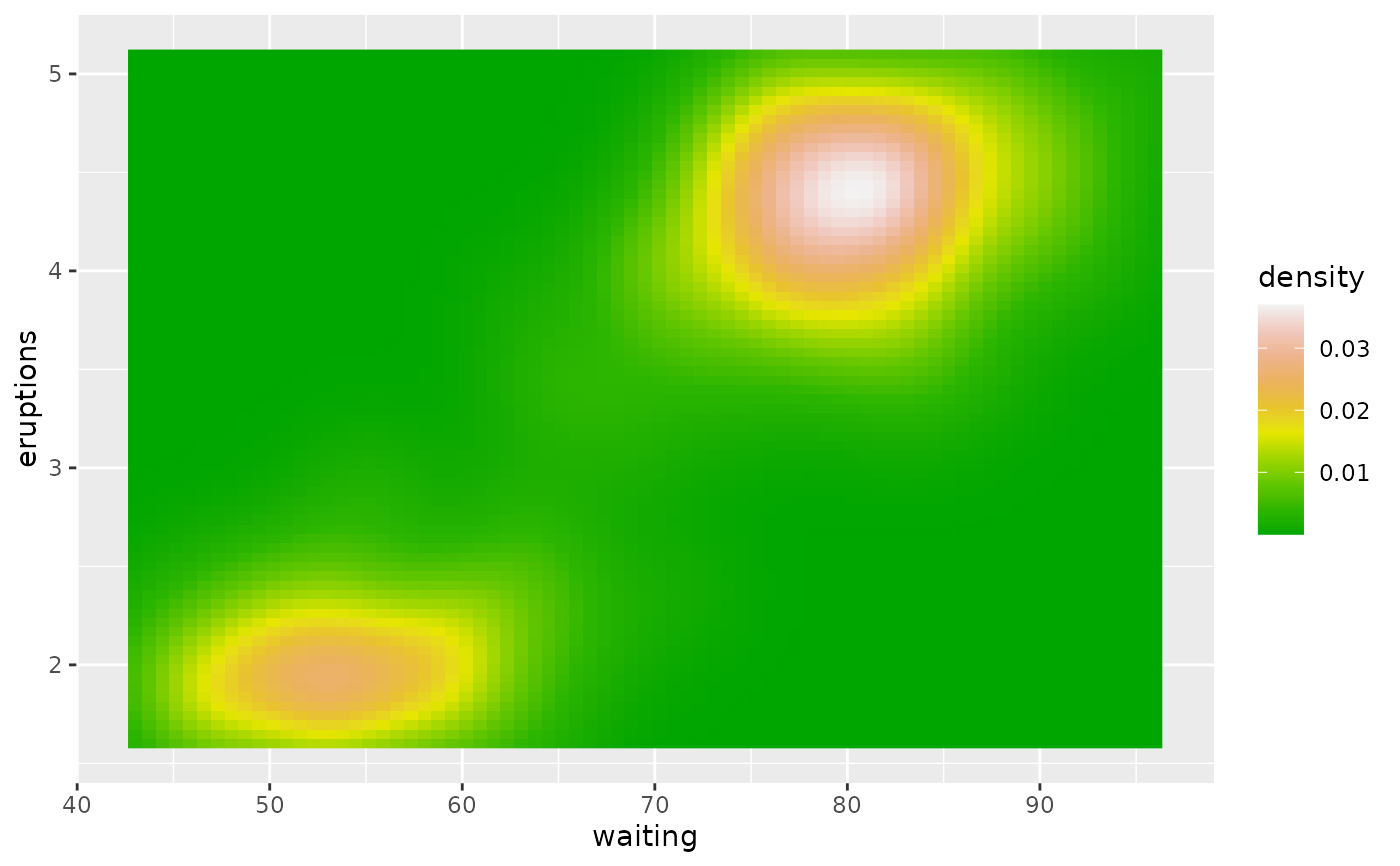# Avoid red-green colour contrasts because ~10% of men have difficulty
# seeing them

# Use na.value = NA to hide missing values but keep the original axis range
ggplot(df_na, aes(x = value, y)) +
geom_bar(aes(fill = z1), stat = "identity") +
scale_fill_gradient(low = "yellow", high = "red", na.value = NA)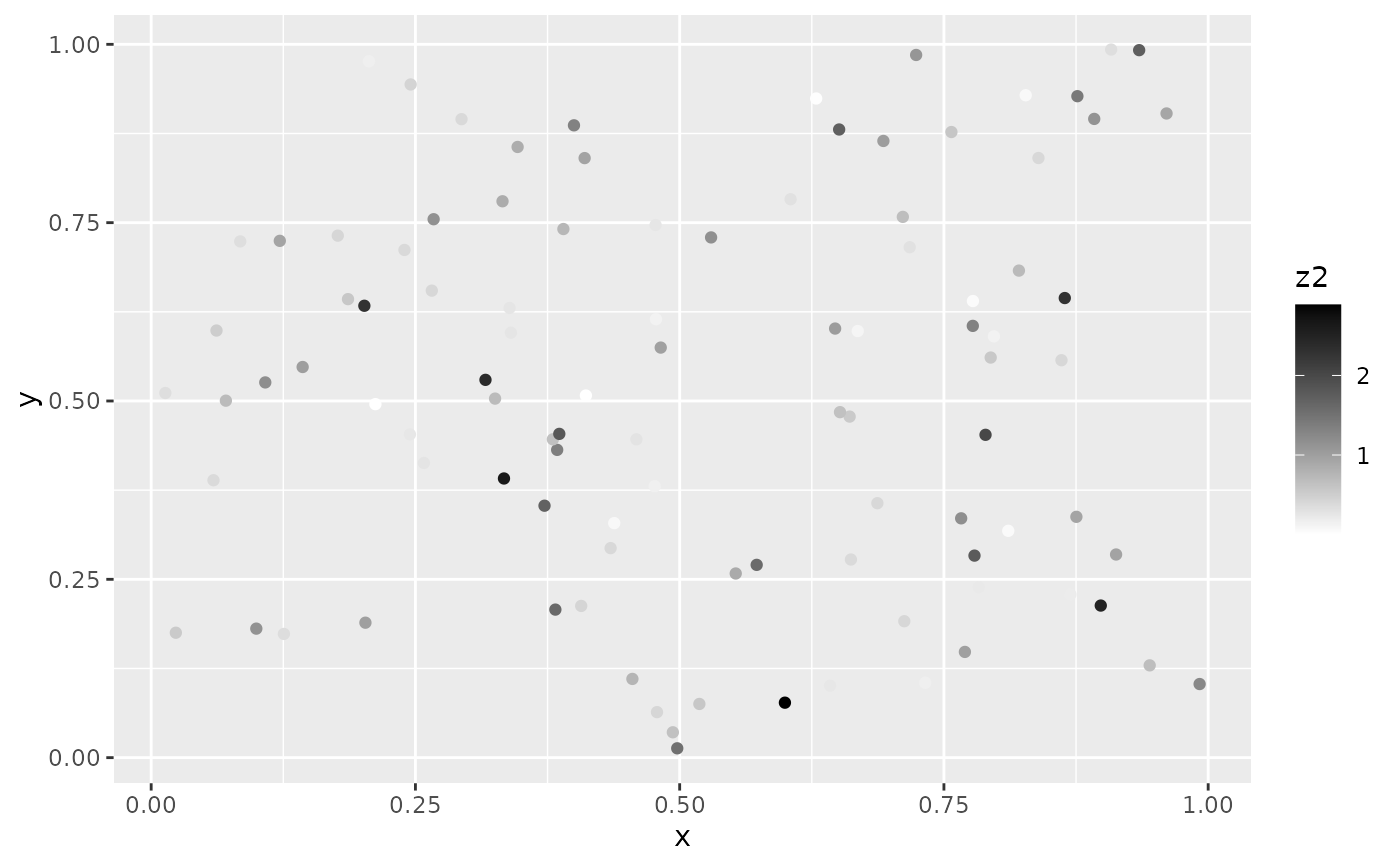ggplot(df_na, aes(x, y)) +
geom_point(aes(colour = z1)) +
scale_colour_gradient(low = "yellow", high = "red", na.value = NA)
#> Warning: Removed 10 rows containing missing values (geom_point).